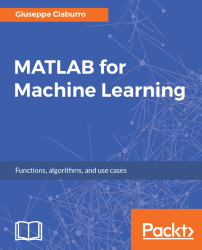•#### MATLAB for Machine Learning#### Overview of this book

MATLAB is the language of choice for many researchers and mathematics experts for machine learning. This book will help you build a foundation in machine learning using MATLAB for beginners. You’ll start by getting your system ready with t he MATLAB environment for machine learning and you’ll see how to easily interact with the Matlab workspace. We’ll then move on to data cleansing, mining and analyzing various data types in machine learning and you’ll see how to display data values on a plot. Next, you’ll get to know about the different types of regression techniques and how to apply them to your data using the MATLAB functions. You’ll understand the basic concepts of neural networks and perform data fitting, pattern recognition, and clustering analysis. Finally, you’ll explore feature selection and extraction techniques for dimensionality reduction for performance improvement. At the end of the book, you will learn to put it all together into real-world cases covering major machine learning algorithms and be comfortable in performing machine learning with MATLAB.
Title PageCreditsForewordwww.PacktPub.comCustomer FeedbackPrefaceFree Chapter
Getting Started with MATLAB Machine LearningImporting and Organizing Data in MATLABFrom Data to Knowledge DiscoveryFinding Relationships between Variables - Regression TechniquesPattern Recognition through Classification AlgorithmsIdentifying Groups of Data Using Clustering MethodsSimulation of Human Thinking - Artificial Neural NetworksImproving the Performance of the Machine Learning Model - Dimensionality ReductionMachine Learning in Practice## Summary

In this chapter, we learned how to perform an accurate regression analysis in a MATLAB environment. First, we explored simple linear regression, how to define it, and how to get an OLS estimation. Then, we looked at several methods of measuring the intercept and slope of a straight line.

Next, we discovered the linear regression model builder; it creates an object inclusive of training data, model description, diagnostic information, and fitted coefficients for a linear regression. Then, we understood how to correctly interpret the results of the simulation and how to reduce outlier effects with robust regression.

So, we explored multiple linear regression techniques; several functions were analyzed to compare the relative results. We learned how to create models with response variables that depend on more than one predictor. Thus, we resolved a multiple linear regression with a categorical predictor example.

With polynomial regression, we approached a model in which some predictors...